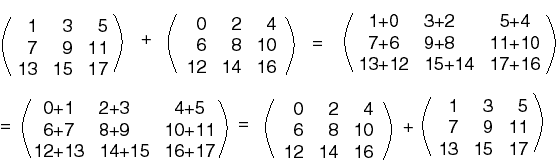Of course, the sum is the same as the non-zero matrix.

# Rules for Matrix Addition

You should be happy with the following rules of matrix addition. In each rule, the matrices are assumed to all have the same dimensions.

• A + B = B + A
• A + 0 = 0 + A = A
• 0 + 0 = 0

These look the same as some rules for addition of real numbers. (Warning!! Not all rules for matrix math look the same as for real number math.)

The first rule says that matrix addition is commutative. This is because ordinary addition is being done on the corresponding elements of the two matrices, and ordinary (real) addition is commutative:### QUESTION 9:

Do you suspect that: (A + B) + C = A + (B + C)